# Excel DAX - Calculated Columns

A calculated column is a column that you add to an existing table in the Data Model of your workbook by means of a DAX formula that defines the column values. Instead of importing the values in the column, you create the calculated column.

You can use the calculated column in a PivotTable, PivotChart, Power PivotTable, Power PivotChart or Power View report just like any other table column.

## Understanding Calculated Columns

The DAX formula used to create a calculated column is like an Excel formula. However, in DAX formula, you cannot create different formulas for different rows in a table. The DAX formula is automatically applied to the entire column.

For example, you can create one calculated column to extract Year from the existing column – Date, with the DAX formula −

```= YEAR ([Date])
```

YEAR is a DAX function and Date is an existing column in the table. As seen, the table name is enclosed in brackets. You will learn more about this in the chapter – DAX Syntax.

When you add a column to a table with this DAX formula, the column values are computed as soon as you create the formula. A new column with the header CalculatedColumn1 filled with Year values will get created.

Column values are recalculated as necessary, such as when the underlying data is refreshed. You can create calculated columns based on existing columns, calculated fields (measures), and other calculated columns.

## Creating a Calculated Column

Consider the Data Model with the Olympics Results as shown in the following screenshot.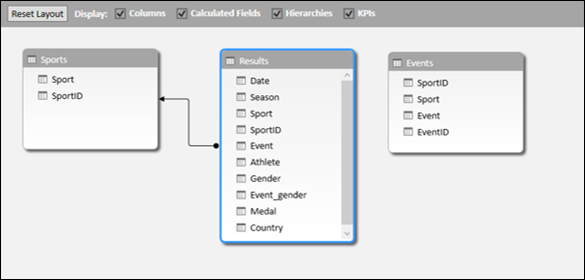• Click the Data View.
• Click the Results tab.

You will be viewing the Results table.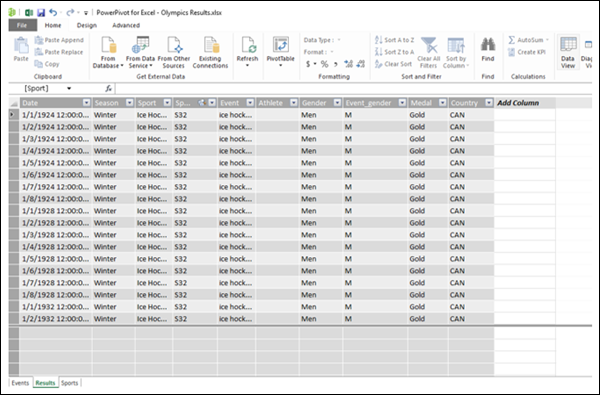As seen in the above screenshot, the rightmost column has the header – Add Column.

• Click the Design tab on the Ribbon.
• Click Add in the Columns group.The pointer will appear in the formula bar. That means you are adding a column with a DAX formula.

• Type =YEAR ([Date]) in the formula bar.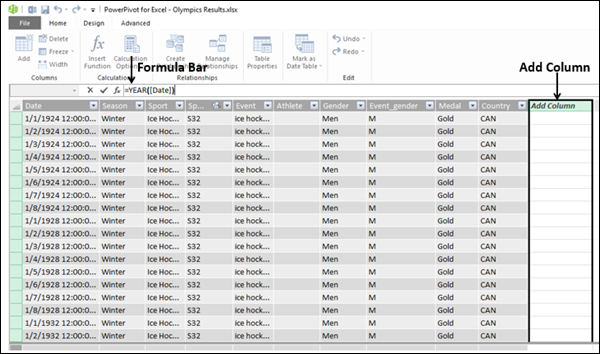As can be seen in the above screenshot, the rightmost column with the header – Add Column is highlighted.

• Press Enter.

It will take a while (few seconds) for the calculations to be done. Please wait.

The new calculated column will get inserted to the left of the rightmost Add Column.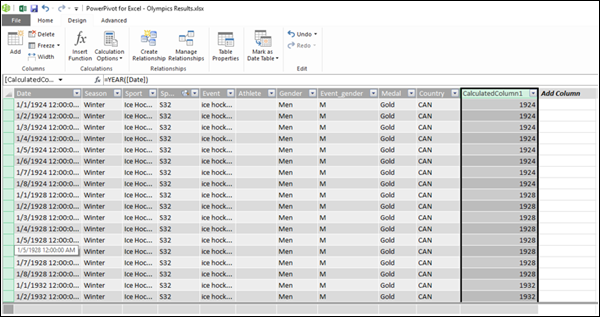As shown in the above screenshot, the newly inserted calculated column is highlighted. Values in the entire column appear as per the DAX formula used. The column header is CalculatedColumn1.

## Renaming the Calculated Column

To rename the calculated column to a meaningful name, do the following −

• Double-click on the column header. The column name will be highlighted.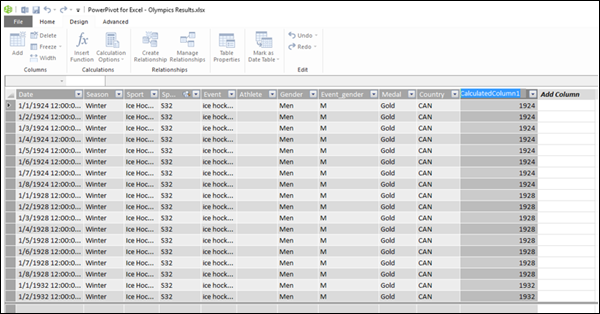• Select the column name.
• Type Year (the new name).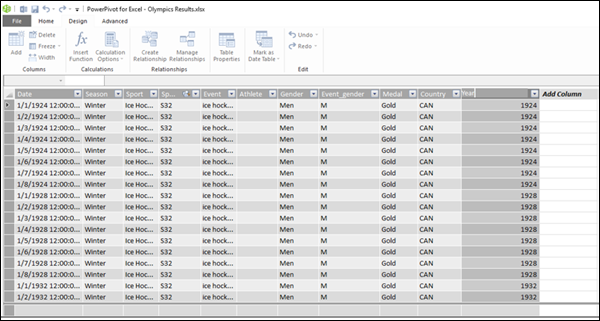As seen in the above screenshot, the name of the calculated column got changed.

You can also rename a calculated column by right-clicking on the column and then clicking on Rename in the dropdown list.

Just make sure that the new name does not conflict with an existing name in the table.

## Checking the Data Type of the Calculated Column

You can check the data type of the calculated column as follows −

• Click the Home tab on the Ribbon.
• Click the Data Type.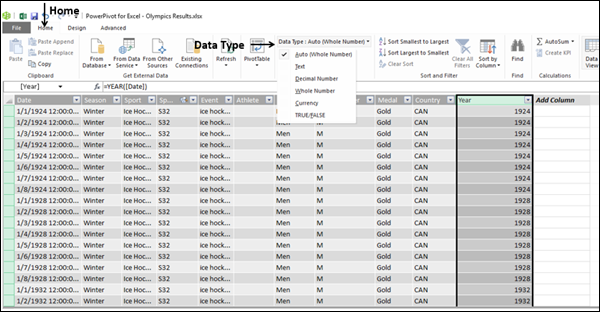As you can see in the above screenshot, the dropdown list has the possible data types for the columns. In this example, the default (Auto) data type, i.e. the Whole Number is selected.

## Errors in Calculated Columns

Errors can occur in the calculated columns for the following reasons −

• Changing or deleting relationships between the tables. This is because the formulas that use columns in those tables will become invalid.

• The formula contains a circular or self-referencing dependency.

## Performance Issues

As seen earlier in the example of Olympics results, the Results table has about 35000 rows of data. Hence, when you created a column with a DAX formula, it had calculated all the 35000+ values in the column at once, for which it took a little while. The Data Model and the tables are meant to handle millions of rows of data. Hence, it can affect the performance when the DAX formula has too many references. You can avoid the performance issues doing the following −

• If your DAX formula contains many complex dependencies, then create it in steps saving the results in new calculated columns, instead of creating a single big formula at once. This enables you to validate the results and assess the performance.

• Calculated columns need to be recalculated when data modifications occur. You can set the recalculation mode to manual, thus saving frequent recalculations. However, if any values in the calculated column are incorrect, the column will be grayed out, until you refresh and recalculate the data.